# Explore model performances¶

This document presents an overview of the results from a variety of model outputs, including further modelling chip probabilities.

There are several aspects of the predictions we’d like to explore and compare across models:

• Overall metrics:

• [x] Kappa score: measure of how many chips the model got right, above what it would have got by pure chance

• [x] Accuracy (micro-F1: general over-simplified measure of how many chips the model got right

• [x] Macro-F1 averaged: mean of each class’ F1

• [x] Macro-F1 weighted: weighted mean of each class’ F1

• Class-based metrics:

• [x] Accuracy: proportion of chips the model got right for each class

• [x] Macro-F1: harmonic mean of precision and recall. Roughly, an overview of how good the model is at predicting each single class

• [x] Confusion matrices: more disaggregated view on predictions. Detailed but not summarising.

• Spatial metrics: compare whether the distribution of predicted labels over space resembles that of the true values

• [x] Moran’s I

• [x] Joint count statistics

• [x] Ripley’s G

• [x] Ripley’s F

import os
import pandas
import geopandas
import numpy as np
import seaborn as sns
import matplotlib.pyplot as plt
import tools_chip_prob_modelling as tools
from copy import deepcopy
from pysal.lib import weights
from pysal.explore import esda

ERROR 1: PROJ: proj_create_from_database: Open of /opt/conda/share/proj failed
/opt/conda/lib/python3.9/site-packages/spaghetti/network.py:36: FutureWarning: The next major release of pysal/spaghetti (2.0.0) will drop support for all libpysal.cg geometries. This change is a first step in refactoring spaghetti that is expected to result in dramatically reduced runtimes for network instantiation and operations. Users currently requiring network and point pattern input as libpysal.cg geometries should prepare for this simply by converting to shapely geometries.
warnings.warn(f"{dep_msg}", FutureWarning)


## Data¶

Here we load the original chip geometries, the true labels and predictions from each model and merge everything into a single geo-table.

• Geometries and true labels

gl = geopandas.read_parquet(
'/home/jovyan/data/spatial_signatures/chip_probs/model_outputs/db.pq',
columns=['label', 'train_all', 'geometry']
)

• Model predictions

Grab all files with model predictions

y_preds_f = [
i for i in os.listdir(
'/home/jovyan/data/spatial_signatures/chip_probs/model_outputs/'
) if '_y_pred.pq' in i
]
y_preds_f

['logite_baseline_wx_y_pred.pq',
'RandomForestClassifier_baseline_wx_y_pred.pq',
'RandomForestClassifier_baseline_y_pred.pq',
'maxprob_baseline_y_pred.pq',
'logite_baseline_y_pred.pq']


Read and store predictions and train/val label

y_preds = pandas.DataFrame(None, index=gl.index)
for f in y_preds_f:
os.path.join('/home/jovyan/data/spatial_signatures/chip_probs/model_outputs/', f)
).set_index(
'id'
).rename(
columns=lambda s: f.replace('_y_pred.pq', '')+'_'+s
)
y_preds = y_preds.join(tab)


Check train/val indices align across models so we can remove them:

vals = [c for c in y_preds.columns if '_Validation' in c]
for i in range(len(vals)-1):
print((y_preds[vals[i]] != y_preds[vals[i+1]]).sum())

0
0
0
0
0
0

• Single table

db = gl.join(y_preds.drop(columns=vals))
db.info()

<class 'geopandas.geodataframe.GeoDataFrame'>
Int64Index: 64409 entries, 4 to 103043
Data columns (total 10 columns):
#   Column                                             Non-Null Count  Dtype
---  ------                                             --------------  -----
0   label                                              64409 non-null  category
1   train_all                                          64409 non-null  bool
2   geometry                                           64409 non-null  geometry
3   logite_baseline_wx_y_pred                          64409 non-null  object
4   RandomForestClassifier_baseline_wx_y_pred          64409 non-null  object
5   RandomForestClassifier_baseline_y_pred             64409 non-null  object
7   maxprob_baseline_y_pred                            64409 non-null  object
9   logite_baseline_y_pred                             64409 non-null  object
dtypes: bool(1), category(1), geometry(1), object(7)
memory usage: 6.6+ MB


## Metrics¶

• Set intuitive order of models

model_names = [
'maxprob_baseline_y_pred',
'logite_baseline_y_pred',
'logite_baseline_wx_y_pred',
'RandomForestClassifier_baseline_y_pred',
'RandomForestClassifier_baseline_wx_y_pred',
]
model_names_short = [
'maxprob_baseline',
'logite',
'logite_wx',
'RF',
'RF_wx',
'HBGB',
'HBGB_wx'
]

• Calculate all metrics by model

metrics = {}
train = db.query('train_all')
val = db.query('~train_all')
for model in model_names:
meta = tools.build_perf(
train['label'],
train[model],
val['label'],
val[model],
tools.class_names
)
metrics[model] = deepcopy(meta)


### Global¶

#### Single scores¶

global_scores = []
for model in metrics:
global_scores.append(pandas.Series(
{i.replace('perf_', '').replace('_val', ''): metrics[model][i] for i in [
'perf_kappa_val',
'perf_model_accuracy_val',
'perf_macro_f1_avg_val',
'perf_macro_f1_w_val'
]},
name=model
))
global_scores = pandas.concat(
global_scores, axis=1
)[
model_names
].rename(
columns=lambda s: s.replace('_y_pred', '')
).T
global_scores

kappa model_accuracy macro_f1_avg macro_f1_w
maxprob_baseline 0.082029 0.240624 0.152201 0.257502
logite_baseline 0.156026 0.418220 0.148086 0.403382
logite_baseline_wx 0.188568 0.426946 0.163392 0.421285
RandomForestClassifier_baseline 0.170341 0.484825 0.167571 0.455233
RandomForestClassifier_baseline_wx 0.262610 0.530728 0.259750 0.505413

#### Confusion matrices¶

Standard version with counts of occurrences as values (left panels) and row standardised (right panels):

f, axs = plt.subplots(4, 4, figsize=(12, 12))
model_loc = [
(0, 0), # Maxprob
(1, 0), # Logite
(1, 1), # Logite Wx
(2, 0), # RF
(2, 1), # RF Wx
(3, 0), # HGBD
(3, 1)  # HGBD Wx
]
# Raw counts #
maxcount = max([np.array(metrics[model]['perf_confusion_val']).max()])
for i, model in enumerate(model_names):
ax = tools.build_cm_plot(
metrics[model]['perf_confusion_val'],
maxcount=maxcount,
ax=axs[model_loc[i]]
)
ax.set_title(model_names_short[i])
axs[0, 1].set_axis_off()
# Std #
for i, model in enumerate(model_names):
xy = model_loc[i]
ax = tools.build_cm_plot(
metrics[model]['perf_confusion_val'],
maxcount=maxcount,
std=True,
ax=axs[xy, xy+2]
)
ax.set_title(model_names_short[i])
axs[0, 3].set_axis_off()
plt.tight_layout()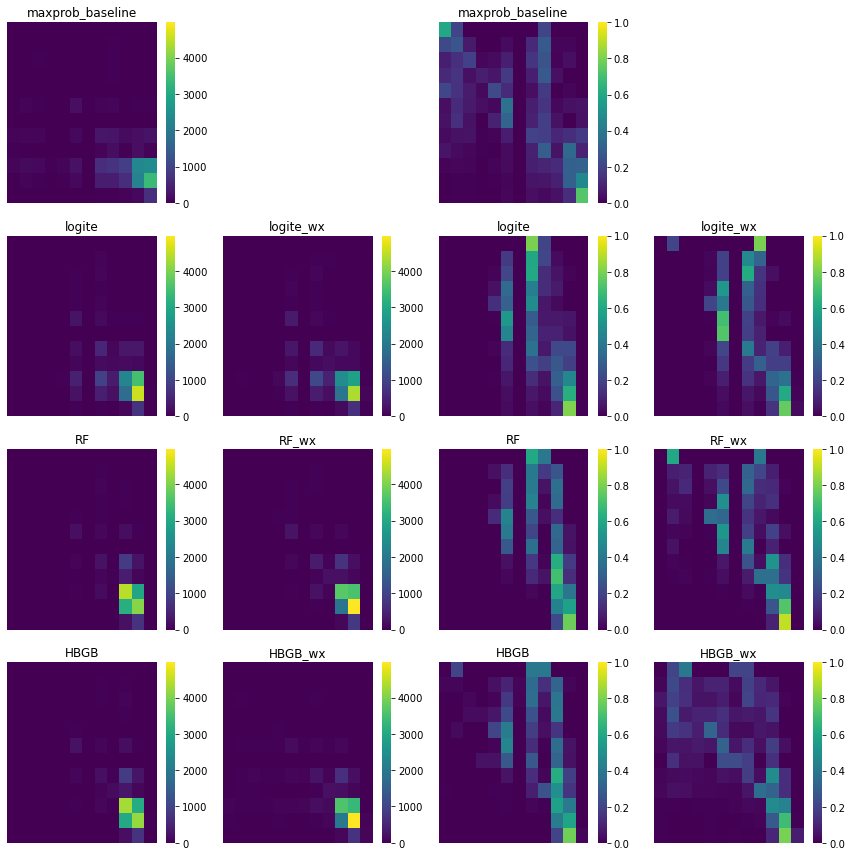### Class-based¶

#### Accuracy¶

wca = []
for i, model in enumerate(model_names):
wca.append(pandas.Series(
metrics[model]['perf_within_class_accuracy_val'],
metrics[model]['meta_class_names'],
name=model_names_short[i]
))
wca = pandas.concat(
wca, axis=1
)[model_names_short].T
wca

urbanity dense_urban_neighbourhoods dense_residential_neighbourhoods connected_residential_neighbourhoods gridded_residential_quarters accessible_suburbia disconnected_suburbia open_sprawl warehouse_park_land urban_buffer countryside_agriculture wild_countryside
maxprob_baseline 0.6 0.263889 0.190476 0.082192 0.223529 0.370526 0.000000 0.195699 0.293907 0.120669 0.303030 0.732816
logite 0.0 0.000000 0.000000 0.000000 0.129412 0.528421 0.000000 0.371326 0.184588 0.300146 0.632074 0.005543
logite_wx 0.0 0.000000 0.000000 0.000000 0.200000 0.698947 0.000000 0.409319 0.297491 0.320324 0.602740 0.025499
RF 0.0 0.000000 0.000000 0.000000 0.117647 0.406316 0.000000 0.156272 0.050179 0.587946 0.562889 0.000000
RF_wx 0.0 0.083333 0.114286 0.000000 0.352941 0.526316 0.000000 0.253047 0.363799 0.488384 0.727411 0.003326
HBGB 0.0 0.013889 0.019048 0.027397 0.200000 0.456842 0.000000 0.128315 0.250896 0.571618 0.578525 0.013304
HBGB_wx 0.0 0.222222 0.133333 0.095890 0.317647 0.309474 0.227273 0.177061 0.354839 0.474977 0.687976 0.080931
h = sns.heatmap(wca, vmin=0, vmax=1, cmap='viridis')
h.set_xticklabels(h.get_xticklabels(), rotation = 45, ha="right")
h.set_title('Within-class Accuracy');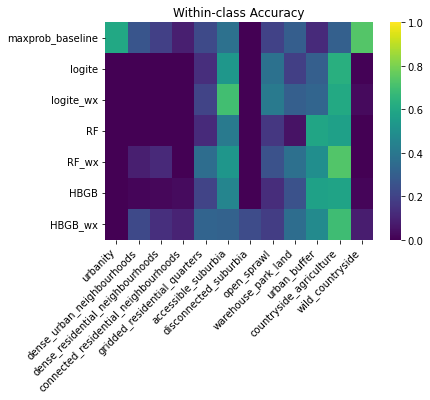#### Macro F1¶

f1 = []
for i, model in enumerate(model_names):
f1.append(pandas.Series(
metrics[model]['perf_f1_val'],
metrics[model]['meta_class_names'],
name=model_names_short[i]
))
f1 = pandas.concat(
f1, axis=1
)[model_names_short].T
f1

urbanity dense_urban_neighbourhoods dense_residential_neighbourhoods connected_residential_neighbourhoods gridded_residential_quarters accessible_suburbia disconnected_suburbia open_sprawl warehouse_park_land urban_buffer countryside_agriculture wild_countryside
maxprob_baseline 0.304762 0.078431 0.358752 0.098765 0.085202 0.000000 0.168889 0.193823 0.195253 0.026316 0.143044 0.173173
logite 0.288009 0.000000 0.555819 0.000000 0.000000 0.000000 0.128655 0.274583 0.372212 0.000000 0.147248 0.010504
logite_wx 0.304029 0.000000 0.568372 0.000000 0.000000 0.000000 0.145923 0.299974 0.389539 0.000000 0.208805 0.044061
RF 0.456805 0.000000 0.533333 0.000000 0.000000 0.000000 0.196078 0.210120 0.527011 0.000000 0.087500 0.000000
RF_wx 0.514403 0.000000 0.613705 0.167832 0.102564 0.000000 0.437956 0.317732 0.516315 0.000000 0.439870 0.006623
HBGB 0.490950 0.052632 0.537680 0.036697 0.025641 0.000000 0.276423 0.190223 0.523844 0.000000 0.354430 0.025668
HBGB_wx 0.371212 0.064815 0.601901 0.108527 0.114695 0.047847 0.189474 0.249874 0.505653 0.000000 0.380769 0.140655
h = sns.heatmap(f1, cmap='viridis')
h.set_xticklabels(h.get_xticklabels(), rotation = 45, ha="right")
h.set_title('F1 Score');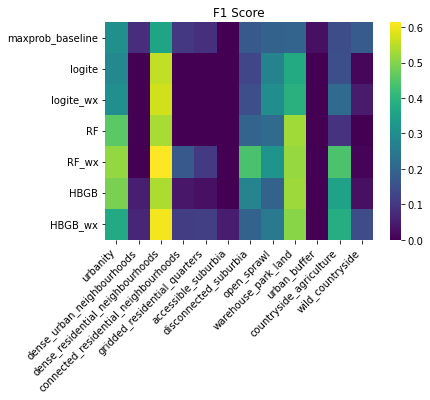### Spatial¶

The goal in this section is to capture how well a set of predictions reproduces the spatial pattern of the original labels. Our first step for this is to consider the labels for a given class. We take those and calculate measures of spatial concentration, either by considering lattice data or a point pattern. In the former case, we compute measures of degree of clustering that treat our data as both continuous (i.e., probability of class occurrence) and discrete. For the latter, we treat instances of a class as a point pattern that is distribtuted across the geography of interest. We then characterise this pattern spatially with a series of explicitly spatial metrics, and calculate those also for the set of predictions from each model we run. Since both the scores for the labels and the predictions is based on the same set of potential locations (i.e. the underlying set of places where a chip can take 1 or 0), the two can be compared directly. Our metrics of interest are thus the difference between the value of a given spatial statistic for the labels, and that for a set of predictions. Smaller quantities will represent greater affinity between the spatial structure of the original labels and that of our predictions. We operationalise this using the validation set.

What we are going to try to do is characterise the degree of clustering of the predictions for each class. For the set of original labels, these are the maps:

f, axs = plt.subplots(4, 3, figsize=(12, 12))
axs = axs.flatten()
for i, c in enumerate(
metrics['maxprob_baseline_y_pred']['meta_class_names']
):
ax = axs[i]
sp_db.assign(v=sp_db['label'] == c).plot(
'v', categorical=True, legend=True, cmap='viridis_r', edgecolor='none', ax=ax
)
ax.set_title(c)
ax.set_axis_off()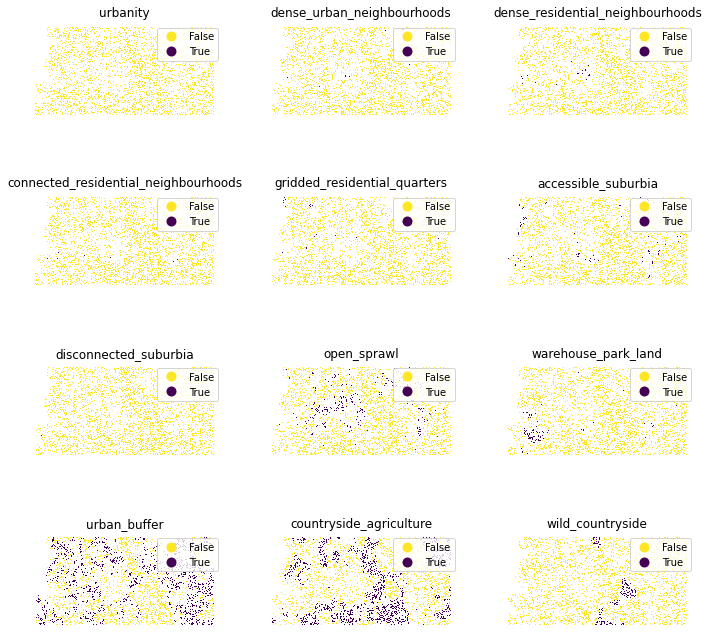We start with only the validation set, as that is the pattern we are interested in characterising.

sp_db = db.query(
'train_all == False'
)


#### Spatial structure (w and coords)¶

For lattice metrics, we conceptualise space in a topological way, for which we build a spatial weights matrix. We work with two different types of topology: a distance band and nearest neighbors.

For the distance band, we ensure every polygon is linked to at least one neighbor by calculating the minimum band we need to apply:

min_thr = weights.min_threshold_distance(
np.array([sp_db.centroid.x, sp_db.centroid.y]).T
)
min_thr

2437.0473938764508


We then build the weights matrix based on that distance:

w_thr = weights.DistanceBand.from_dataframe(sp_db, min_thr)


This produces a set of cardinalities we can explore with an eye to match similarly with the $$k$$-nearest neighbors weights later.

pandas.Series(w_thr.cardinalities).plot.hist(bins=68, figsize=(9, 3));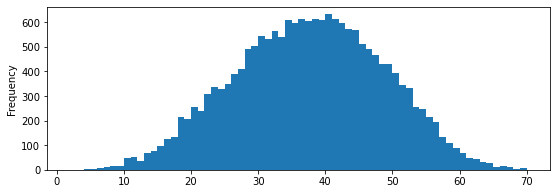We then use $$k=35$$ to build the nearest neighbor weights:

w_knn = weights.KNN.from_dataframe(sp_db, k=35)


The point coordinates of each chip are a more straightforward task. We store them as a ndarray as that’s how we’ll need them later:

xys = sp_db.centroid
xys = np.array([xys.x, xys.y]).T


#### Metric computation¶

To accelerate computations across different cores, we set it up in Dask:

from dask import bag as dbag

client = Client(LocalCluster(n_workers=8))

Numba: Attempted to fork from a non-main thread, the TBB library may be in an invalid state in the child process.
Numba: Attempted to fork from a non-main thread, the TBB library may be in an invalid state in the child process.
Numba: Attempted to fork from a non-main thread, the TBB library may be in an invalid state in the child process.
Numba: Attempted to fork from a non-main thread, the TBB library may be in an invalid state in the child process.
Numba: Attempted to fork from a non-main thread, the TBB library may be in an invalid state in the child process.
Numba: Attempted to fork from a non-main thread, the TBB library may be in an invalid state in the child process.
Numba: Attempted to fork from a non-main thread, the TBB library may be in an invalid state in the child process.
Numba: Attempted to fork from a non-main thread, the TBB library may be in an invalid state in the child process.
Numba: Attempted to fork from a non-main thread, the TBB library may be in an invalid state in the child process.
2022-08-07 14:07:35,668 - distributed.diskutils - INFO - Found stale lock file and directory '/home/jovyan/work/code/signature_ai/ai_experiments/dask-worker-space/worker-uep79n9d', purging
2022-08-07 14:07:35,668 - distributed.diskutils - INFO - Found stale lock file and directory '/home/jovyan/work/code/signature_ai/ai_experiments/dask-worker-space/worker-63ansyiy', purging
2022-08-07 14:07:35,668 - distributed.diskutils - INFO - Found stale lock file and directory '/home/jovyan/work/code/signature_ai/ai_experiments/dask-worker-space/worker-b38pqrvd', purging

client.restart()


And span the computations of all the statistics across different cores:

from importlib import reload

%%time
wd = {'thr': w_thr, 'knn': w_knn}
#     y         w    xys       Name
sp_db[model], wd, xys, model
) for model in model_names+['label']]

tools.spatial_perf
).compute())

ERROR 1: PROJ: proj_create_from_database: Open of /opt/conda/share/proj failed
ERROR 1: PROJ: proj_create_from_database: Open of /opt/conda/share/proj failed
ERROR 1: PROJ: proj_create_from_database: Open of /opt/conda/share/proj failed
ERROR 1: PROJ: proj_create_from_database: Open of /opt/conda/share/proj failed
ERROR 1: PROJ: proj_create_from_database: Open of /opt/conda/share/proj failed
ERROR 1: PROJ: proj_create_from_database: Open of /opt/conda/share/proj failed
ERROR 1: PROJ: proj_create_from_database: Open of /opt/conda/share/proj failed
ERROR 1: PROJ: proj_create_from_database: Open of /opt/conda/share/proj failed
/opt/conda/lib/python3.9/site-packages/esda/moran.py:193: RuntimeWarning: divide by zero encountered in double_scalars
self.z_sim = (self.I - self.EI_sim) / self.seI_sim
/opt/conda/lib/python3.9/site-packages/esda/moran.py:193: RuntimeWarning: divide by zero encountered in double_scalars
self.z_sim = (self.I - self.EI_sim) / self.seI_sim
/opt/conda/lib/python3.9/site-packages/esda/moran.py:193: RuntimeWarning: divide by zero encountered in double_scalars
self.z_sim = (self.I - self.EI_sim) / self.seI_sim
/opt/conda/lib/python3.9/site-packages/esda/moran.py:193: RuntimeWarning: divide by zero encountered in double_scalars
self.z_sim = (self.I - self.EI_sim) / self.seI_sim
/opt/conda/lib/python3.9/site-packages/esda/moran.py:193: RuntimeWarning: divide by zero encountered in double_scalars
self.z_sim = (self.I - self.EI_sim) / self.seI_sim
/opt/conda/lib/python3.9/site-packages/esda/moran.py:193: RuntimeWarning: divide by zero encountered in double_scalars
self.z_sim = (self.I - self.EI_sim) / self.seI_sim
/opt/conda/lib/python3.9/site-packages/esda/moran.py:193: RuntimeWarning: divide by zero encountered in double_scalars
self.z_sim = (self.I - self.EI_sim) / self.seI_sim
/opt/conda/lib/python3.9/site-packages/esda/moran.py:193: RuntimeWarning: divide by zero encountered in double_scalars
self.z_sim = (self.I - self.EI_sim) / self.seI_sim
/opt/conda/lib/python3.9/site-packages/esda/moran.py:193: RuntimeWarning: divide by zero encountered in double_scalars
self.z_sim = (self.I - self.EI_sim) / self.seI_sim
/opt/conda/lib/python3.9/site-packages/esda/moran.py:193: RuntimeWarning: divide by zero encountered in double_scalars
self.z_sim = (self.I - self.EI_sim) / self.seI_sim
/opt/conda/lib/python3.9/site-packages/esda/moran.py:193: RuntimeWarning: divide by zero encountered in double_scalars
self.z_sim = (self.I - self.EI_sim) / self.seI_sim
/opt/conda/lib/python3.9/site-packages/esda/moran.py:193: RuntimeWarning: divide by zero encountered in double_scalars
self.z_sim = (self.I - self.EI_sim) / self.seI_sim
/opt/conda/lib/python3.9/site-packages/esda/moran.py:193: RuntimeWarning: divide by zero encountered in double_scalars
self.z_sim = (self.I - self.EI_sim) / self.seI_sim
/opt/conda/lib/python3.9/site-packages/esda/moran.py:193: RuntimeWarning: divide by zero encountered in double_scalars
self.z_sim = (self.I - self.EI_sim) / self.seI_sim
/opt/conda/lib/python3.9/site-packages/esda/moran.py:193: RuntimeWarning: divide by zero encountered in double_scalars
self.z_sim = (self.I - self.EI_sim) / self.seI_sim
/opt/conda/lib/python3.9/site-packages/esda/moran.py:193: RuntimeWarning: divide by zero encountered in double_scalars
self.z_sim = (self.I - self.EI_sim) / self.seI_sim
/opt/conda/lib/python3.9/site-packages/esda/moran.py:193: RuntimeWarning: divide by zero encountered in double_scalars
self.z_sim = (self.I - self.EI_sim) / self.seI_sim
/opt/conda/lib/python3.9/site-packages/esda/moran.py:193: RuntimeWarning: divide by zero encountered in double_scalars
self.z_sim = (self.I - self.EI_sim) / self.seI_sim
/opt/conda/lib/python3.9/site-packages/esda/moran.py:193: RuntimeWarning: divide by zero encountered in double_scalars
self.z_sim = (self.I - self.EI_sim) / self.seI_sim
/opt/conda/lib/python3.9/site-packages/esda/moran.py:193: RuntimeWarning: divide by zero encountered in double_scalars
self.z_sim = (self.I - self.EI_sim) / self.seI_sim
/opt/conda/lib/python3.9/site-packages/esda/moran.py:193: RuntimeWarning: divide by zero encountered in double_scalars
self.z_sim = (self.I - self.EI_sim) / self.seI_sim
/opt/conda/lib/python3.9/site-packages/esda/moran.py:193: RuntimeWarning: divide by zero encountered in double_scalars
self.z_sim = (self.I - self.EI_sim) / self.seI_sim
/opt/conda/lib/python3.9/site-packages/esda/moran.py:193: RuntimeWarning: divide by zero encountered in double_scalars
self.z_sim = (self.I - self.EI_sim) / self.seI_sim
/opt/conda/lib/python3.9/site-packages/esda/moran.py:193: RuntimeWarning: divide by zero encountered in double_scalars
self.z_sim = (self.I - self.EI_sim) / self.seI_sim
/opt/conda/lib/python3.9/site-packages/esda/moran.py:193: RuntimeWarning: divide by zero encountered in double_scalars
self.z_sim = (self.I - self.EI_sim) / self.seI_sim
/opt/conda/lib/python3.9/site-packages/esda/moran.py:193: RuntimeWarning: divide by zero encountered in double_scalars
self.z_sim = (self.I - self.EI_sim) / self.seI_sim
/opt/conda/lib/python3.9/site-packages/esda/moran.py:193: RuntimeWarning: divide by zero encountered in double_scalars
self.z_sim = (self.I - self.EI_sim) / self.seI_sim
/opt/conda/lib/python3.9/site-packages/esda/moran.py:193: RuntimeWarning: divide by zero encountered in double_scalars
self.z_sim = (self.I - self.EI_sim) / self.seI_sim
/opt/conda/lib/python3.9/site-packages/esda/moran.py:193: RuntimeWarning: divide by zero encountered in double_scalars
self.z_sim = (self.I - self.EI_sim) / self.seI_sim
/opt/conda/lib/python3.9/site-packages/esda/moran.py:193: RuntimeWarning: divide by zero encountered in double_scalars
self.z_sim = (self.I - self.EI_sim) / self.seI_sim
/opt/conda/lib/python3.9/site-packages/esda/moran.py:193: RuntimeWarning: divide by zero encountered in double_scalars
self.z_sim = (self.I - self.EI_sim) / self.seI_sim
/opt/conda/lib/python3.9/site-packages/esda/moran.py:193: RuntimeWarning: divide by zero encountered in double_scalars
self.z_sim = (self.I - self.EI_sim) / self.seI_sim
/opt/conda/lib/python3.9/site-packages/esda/moran.py:193: RuntimeWarning: divide by zero encountered in double_scalars
self.z_sim = (self.I - self.EI_sim) / self.seI_sim
/opt/conda/lib/python3.9/site-packages/esda/moran.py:193: RuntimeWarning: divide by zero encountered in double_scalars
self.z_sim = (self.I - self.EI_sim) / self.seI_sim
/opt/conda/lib/python3.9/site-packages/esda/moran.py:193: RuntimeWarning: divide by zero encountered in double_scalars
self.z_sim = (self.I - self.EI_sim) / self.seI_sim
/opt/conda/lib/python3.9/site-packages/esda/moran.py:193: RuntimeWarning: divide by zero encountered in double_scalars
self.z_sim = (self.I - self.EI_sim) / self.seI_sim
/opt/conda/lib/python3.9/site-packages/esda/moran.py:193: RuntimeWarning: divide by zero encountered in double_scalars
self.z_sim = (self.I - self.EI_sim) / self.seI_sim
/opt/conda/lib/python3.9/site-packages/esda/moran.py:193: RuntimeWarning: divide by zero encountered in double_scalars
self.z_sim = (self.I - self.EI_sim) / self.seI_sim
/opt/conda/lib/python3.9/site-packages/esda/moran.py:193: RuntimeWarning: divide by zero encountered in double_scalars
self.z_sim = (self.I - self.EI_sim) / self.seI_sim
/opt/conda/lib/python3.9/site-packages/esda/moran.py:193: RuntimeWarning: divide by zero encountered in double_scalars
self.z_sim = (self.I - self.EI_sim) / self.seI_sim
/opt/conda/lib/python3.9/site-packages/esda/moran.py:193: RuntimeWarning: divide by zero encountered in double_scalars
self.z_sim = (self.I - self.EI_sim) / self.seI_sim
/opt/conda/lib/python3.9/site-packages/esda/moran.py:193: RuntimeWarning: divide by zero encountered in double_scalars
self.z_sim = (self.I - self.EI_sim) / self.seI_sim
/opt/conda/lib/python3.9/site-packages/esda/moran.py:193: RuntimeWarning: divide by zero encountered in double_scalars
self.z_sim = (self.I - self.EI_sim) / self.seI_sim
/opt/conda/lib/python3.9/site-packages/esda/moran.py:193: RuntimeWarning: divide by zero encountered in double_scalars
self.z_sim = (self.I - self.EI_sim) / self.seI_sim
/opt/conda/lib/python3.9/site-packages/esda/moran.py:193: RuntimeWarning: divide by zero encountered in double_scalars
self.z_sim = (self.I - self.EI_sim) / self.seI_sim
/opt/conda/lib/python3.9/site-packages/esda/moran.py:193: RuntimeWarning: divide by zero encountered in double_scalars
self.z_sim = (self.I - self.EI_sim) / self.seI_sim
/opt/conda/lib/python3.9/site-packages/esda/moran.py:193: RuntimeWarning: divide by zero encountered in double_scalars
self.z_sim = (self.I - self.EI_sim) / self.seI_sim
/opt/conda/lib/python3.9/site-packages/esda/moran.py:193: RuntimeWarning: divide by zero encountered in double_scalars
self.z_sim = (self.I - self.EI_sim) / self.seI_sim
/opt/conda/lib/python3.9/site-packages/esda/moran.py:193: RuntimeWarning: divide by zero encountered in double_scalars
self.z_sim = (self.I - self.EI_sim) / self.seI_sim
/opt/conda/lib/python3.9/site-packages/esda/moran.py:193: RuntimeWarning: divide by zero encountered in double_scalars
self.z_sim = (self.I - self.EI_sim) / self.seI_sim
/opt/conda/lib/python3.9/site-packages/esda/moran.py:193: RuntimeWarning: divide by zero encountered in double_scalars
self.z_sim = (self.I - self.EI_sim) / self.seI_sim
/opt/conda/lib/python3.9/site-packages/esda/moran.py:193: RuntimeWarning: divide by zero encountered in double_scalars
self.z_sim = (self.I - self.EI_sim) / self.seI_sim
/opt/conda/lib/python3.9/site-packages/esda/moran.py:193: RuntimeWarning: divide by zero encountered in double_scalars
self.z_sim = (self.I - self.EI_sim) / self.seI_sim
/opt/conda/lib/python3.9/site-packages/esda/moran.py:193: RuntimeWarning: divide by zero encountered in double_scalars
self.z_sim = (self.I - self.EI_sim) / self.seI_sim
/opt/conda/lib/python3.9/site-packages/esda/moran.py:193: RuntimeWarning: divide by zero encountered in double_scalars
self.z_sim = (self.I - self.EI_sim) / self.seI_sim
/opt/conda/lib/python3.9/site-packages/esda/moran.py:193: RuntimeWarning: divide by zero encountered in double_scalars
self.z_sim = (self.I - self.EI_sim) / self.seI_sim
/opt/conda/lib/python3.9/site-packages/esda/moran.py:193: RuntimeWarning: divide by zero encountered in double_scalars
self.z_sim = (self.I - self.EI_sim) / self.seI_sim
/opt/conda/lib/python3.9/site-packages/esda/moran.py:193: RuntimeWarning: divide by zero encountered in double_scalars
self.z_sim = (self.I - self.EI_sim) / self.seI_sim
/opt/conda/lib/python3.9/site-packages/esda/moran.py:193: RuntimeWarning: divide by zero encountered in double_scalars
self.z_sim = (self.I - self.EI_sim) / self.seI_sim
/opt/conda/lib/python3.9/site-packages/esda/moran.py:193: RuntimeWarning: divide by zero encountered in double_scalars
self.z_sim = (self.I - self.EI_sim) / self.seI_sim
/opt/conda/lib/python3.9/site-packages/esda/moran.py:193: RuntimeWarning: divide by zero encountered in double_scalars
self.z_sim = (self.I - self.EI_sim) / self.seI_sim
/opt/conda/lib/python3.9/site-packages/esda/moran.py:193: RuntimeWarning: divide by zero encountered in double_scalars
self.z_sim = (self.I - self.EI_sim) / self.seI_sim
/opt/conda/lib/python3.9/site-packages/esda/moran.py:193: RuntimeWarning: divide by zero encountered in double_scalars
self.z_sim = (self.I - self.EI_sim) / self.seI_sim
/opt/conda/lib/python3.9/site-packages/esda/moran.py:193: RuntimeWarning: divide by zero encountered in double_scalars
self.z_sim = (self.I - self.EI_sim) / self.seI_sim
/opt/conda/lib/python3.9/site-packages/esda/moran.py:193: RuntimeWarning: divide by zero encountered in double_scalars
self.z_sim = (self.I - self.EI_sim) / self.seI_sim
/opt/conda/lib/python3.9/site-packages/esda/moran.py:193: RuntimeWarning: divide by zero encountered in double_scalars
self.z_sim = (self.I - self.EI_sim) / self.seI_sim
/opt/conda/lib/python3.9/site-packages/esda/moran.py:193: RuntimeWarning: divide by zero encountered in double_scalars
self.z_sim = (self.I - self.EI_sim) / self.seI_sim
/opt/conda/lib/python3.9/site-packages/esda/moran.py:193: RuntimeWarning: divide by zero encountered in double_scalars
self.z_sim = (self.I - self.EI_sim) / self.seI_sim
/opt/conda/lib/python3.9/site-packages/esda/moran.py:193: RuntimeWarning: divide by zero encountered in double_scalars
self.z_sim = (self.I - self.EI_sim) / self.seI_sim
/opt/conda/lib/python3.9/site-packages/esda/moran.py:193: RuntimeWarning: divide by zero encountered in double_scalars
self.z_sim = (self.I - self.EI_sim) / self.seI_sim
/opt/conda/lib/python3.9/site-packages/esda/moran.py:193: RuntimeWarning: divide by zero encountered in double_scalars
self.z_sim = (self.I - self.EI_sim) / self.seI_sim
/opt/conda/lib/python3.9/site-packages/esda/moran.py:193: RuntimeWarning: divide by zero encountered in double_scalars
self.z_sim = (self.I - self.EI_sim) / self.seI_sim
/opt/conda/lib/python3.9/site-packages/esda/moran.py:193: RuntimeWarning: divide by zero encountered in double_scalars
self.z_sim = (self.I - self.EI_sim) / self.seI_sim
/opt/conda/lib/python3.9/site-packages/esda/moran.py:193: RuntimeWarning: divide by zero encountered in double_scalars
self.z_sim = (self.I - self.EI_sim) / self.seI_sim
/opt/conda/lib/python3.9/site-packages/esda/moran.py:193: RuntimeWarning: divide by zero encountered in double_scalars
self.z_sim = (self.I - self.EI_sim) / self.seI_sim
/opt/conda/lib/python3.9/site-packages/esda/moran.py:193: RuntimeWarning: divide by zero encountered in double_scalars
self.z_sim = (self.I - self.EI_sim) / self.seI_sim
/opt/conda/lib/python3.9/site-packages/esda/moran.py:193: RuntimeWarning: divide by zero encountered in double_scalars
self.z_sim = (self.I - self.EI_sim) / self.seI_sim
/opt/conda/lib/python3.9/site-packages/esda/moran.py:193: RuntimeWarning: divide by zero encountered in double_scalars
self.z_sim = (self.I - self.EI_sim) / self.seI_sim
/opt/conda/lib/python3.9/site-packages/esda/moran.py:193: RuntimeWarning: divide by zero encountered in double_scalars
self.z_sim = (self.I - self.EI_sim) / self.seI_sim
/opt/conda/lib/python3.9/site-packages/esda/moran.py:193: RuntimeWarning: divide by zero encountered in double_scalars
self.z_sim = (self.I - self.EI_sim) / self.seI_sim
/opt/conda/lib/python3.9/site-packages/esda/moran.py:193: RuntimeWarning: divide by zero encountered in double_scalars
self.z_sim = (self.I - self.EI_sim) / self.seI_sim
/opt/conda/lib/python3.9/site-packages/esda/moran.py:193: RuntimeWarning: divide by zero encountered in double_scalars
self.z_sim = (self.I - self.EI_sim) / self.seI_sim
/opt/conda/lib/python3.9/site-packages/esda/moran.py:193: RuntimeWarning: divide by zero encountered in double_scalars
self.z_sim = (self.I - self.EI_sim) / self.seI_sim
/opt/conda/lib/python3.9/site-packages/esda/moran.py:193: RuntimeWarning: divide by zero encountered in double_scalars
self.z_sim = (self.I - self.EI_sim) / self.seI_sim
/opt/conda/lib/python3.9/site-packages/esda/moran.py:193: RuntimeWarning: divide by zero encountered in double_scalars
self.z_sim = (self.I - self.EI_sim) / self.seI_sim
/opt/conda/lib/python3.9/site-packages/esda/moran.py:193: RuntimeWarning: divide by zero encountered in double_scalars
self.z_sim = (self.I - self.EI_sim) / self.seI_sim
/opt/conda/lib/python3.9/site-packages/esda/moran.py:193: RuntimeWarning: divide by zero encountered in double_scalars
self.z_sim = (self.I - self.EI_sim) / self.seI_sim
/opt/conda/lib/python3.9/site-packages/esda/moran.py:193: RuntimeWarning: divide by zero encountered in double_scalars
self.z_sim = (self.I - self.EI_sim) / self.seI_sim
/opt/conda/lib/python3.9/site-packages/esda/moran.py:193: RuntimeWarning: divide by zero encountered in double_scalars
self.z_sim = (self.I - self.EI_sim) / self.seI_sim
/opt/conda/lib/python3.9/site-packages/esda/moran.py:193: RuntimeWarning: divide by zero encountered in double_scalars
self.z_sim = (self.I - self.EI_sim) / self.seI_sim
/opt/conda/lib/python3.9/site-packages/esda/moran.py:193: RuntimeWarning: divide by zero encountered in double_scalars
self.z_sim = (self.I - self.EI_sim) / self.seI_sim
/opt/conda/lib/python3.9/site-packages/esda/moran.py:193: RuntimeWarning: divide by zero encountered in double_scalars
self.z_sim = (self.I - self.EI_sim) / self.seI_sim
/opt/conda/lib/python3.9/site-packages/esda/moran.py:193: RuntimeWarning: divide by zero encountered in double_scalars
self.z_sim = (self.I - self.EI_sim) / self.seI_sim
/opt/conda/lib/python3.9/site-packages/esda/moran.py:193: RuntimeWarning: divide by zero encountered in double_scalars
self.z_sim = (self.I - self.EI_sim) / self.seI_sim
/opt/conda/lib/python3.9/site-packages/esda/moran.py:193: RuntimeWarning: divide by zero encountered in double_scalars
self.z_sim = (self.I - self.EI_sim) / self.seI_sim
/opt/conda/lib/python3.9/site-packages/esda/moran.py:193: RuntimeWarning: divide by zero encountered in double_scalars
self.z_sim = (self.I - self.EI_sim) / self.seI_sim
/opt/conda/lib/python3.9/site-packages/esda/moran.py:193: RuntimeWarning: divide by zero encountered in double_scalars
self.z_sim = (self.I - self.EI_sim) / self.seI_sim
/opt/conda/lib/python3.9/site-packages/esda/moran.py:193: RuntimeWarning: divide by zero encountered in double_scalars
self.z_sim = (self.I - self.EI_sim) / self.seI_sim
/opt/conda/lib/python3.9/site-packages/esda/moran.py:193: RuntimeWarning: divide by zero encountered in double_scalars
self.z_sim = (self.I - self.EI_sim) / self.seI_sim
/opt/conda/lib/python3.9/site-packages/esda/moran.py:193: RuntimeWarning: divide by zero encountered in double_scalars
self.z_sim = (self.I - self.EI_sim) / self.seI_sim
/opt/conda/lib/python3.9/site-packages/esda/moran.py:193: RuntimeWarning: divide by zero encountered in double_scalars
self.z_sim = (self.I - self.EI_sim) / self.seI_sim
/opt/conda/lib/python3.9/site-packages/esda/moran.py:193: RuntimeWarning: divide by zero encountered in double_scalars
self.z_sim = (self.I - self.EI_sim) / self.seI_sim
/opt/conda/lib/python3.9/site-packages/esda/moran.py:193: RuntimeWarning: divide by zero encountered in double_scalars
self.z_sim = (self.I - self.EI_sim) / self.seI_sim
/opt/conda/lib/python3.9/site-packages/esda/moran.py:193: RuntimeWarning: divide by zero encountered in double_scalars
self.z_sim = (self.I - self.EI_sim) / self.seI_sim
/opt/conda/lib/python3.9/site-packages/esda/moran.py:193: RuntimeWarning: divide by zero encountered in double_scalars
self.z_sim = (self.I - self.EI_sim) / self.seI_sim
/opt/conda/lib/python3.9/site-packages/esda/moran.py:193: RuntimeWarning: divide by zero encountered in double_scalars
self.z_sim = (self.I - self.EI_sim) / self.seI_sim
/opt/conda/lib/python3.9/site-packages/esda/moran.py:193: RuntimeWarning: divide by zero encountered in double_scalars
self.z_sim = (self.I - self.EI_sim) / self.seI_sim
/opt/conda/lib/python3.9/site-packages/esda/moran.py:193: RuntimeWarning: divide by zero encountered in double_scalars
self.z_sim = (self.I - self.EI_sim) / self.seI_sim
/opt/conda/lib/python3.9/site-packages/esda/moran.py:193: RuntimeWarning: divide by zero encountered in double_scalars
self.z_sim = (self.I - self.EI_sim) / self.seI_sim
/opt/conda/lib/python3.9/site-packages/esda/moran.py:193: RuntimeWarning: divide by zero encountered in double_scalars
self.z_sim = (self.I - self.EI_sim) / self.seI_sim
/opt/conda/lib/python3.9/site-packages/esda/moran.py:193: RuntimeWarning: divide by zero encountered in double_scalars
self.z_sim = (self.I - self.EI_sim) / self.seI_sim
/opt/conda/lib/python3.9/site-packages/esda/moran.py:193: RuntimeWarning: divide by zero encountered in double_scalars
self.z_sim = (self.I - self.EI_sim) / self.seI_sim
/opt/conda/lib/python3.9/site-packages/esda/moran.py:193: RuntimeWarning: divide by zero encountered in double_scalars
self.z_sim = (self.I - self.EI_sim) / self.seI_sim
/opt/conda/lib/python3.9/site-packages/esda/moran.py:193: RuntimeWarning: divide by zero encountered in double_scalars
self.z_sim = (self.I - self.EI_sim) / self.seI_sim
/opt/conda/lib/python3.9/site-packages/esda/moran.py:193: RuntimeWarning: divide by zero encountered in double_scalars
self.z_sim = (self.I - self.EI_sim) / self.seI_sim
/opt/conda/lib/python3.9/site-packages/esda/moran.py:193: RuntimeWarning: divide by zero encountered in double_scalars
self.z_sim = (self.I - self.EI_sim) / self.seI_sim
/opt/conda/lib/python3.9/site-packages/esda/moran.py:193: RuntimeWarning: divide by zero encountered in double_scalars
self.z_sim = (self.I - self.EI_sim) / self.seI_sim
/opt/conda/lib/python3.9/site-packages/esda/moran.py:193: RuntimeWarning: divide by zero encountered in double_scalars
self.z_sim = (self.I - self.EI_sim) / self.seI_sim
/opt/conda/lib/python3.9/site-packages/esda/moran.py:193: RuntimeWarning: divide by zero encountered in double_scalars
self.z_sim = (self.I - self.EI_sim) / self.seI_sim
/opt/conda/lib/python3.9/site-packages/esda/moran.py:193: RuntimeWarning: divide by zero encountered in double_scalars
self.z_sim = (self.I - self.EI_sim) / self.seI_sim
/opt/conda/lib/python3.9/site-packages/esda/moran.py:193: RuntimeWarning: divide by zero encountered in double_scalars
self.z_sim = (self.I - self.EI_sim) / self.seI_sim
/opt/conda/lib/python3.9/site-packages/esda/moran.py:193: RuntimeWarning: divide by zero encountered in double_scalars
self.z_sim = (self.I - self.EI_sim) / self.seI_sim
/opt/conda/lib/python3.9/site-packages/esda/moran.py:193: RuntimeWarning: divide by zero encountered in double_scalars
self.z_sim = (self.I - self.EI_sim) / self.seI_sim
/opt/conda/lib/python3.9/site-packages/esda/moran.py:193: RuntimeWarning: divide by zero encountered in double_scalars
self.z_sim = (self.I - self.EI_sim) / self.seI_sim
/opt/conda/lib/python3.9/site-packages/esda/moran.py:193: RuntimeWarning: divide by zero encountered in double_scalars
self.z_sim = (self.I - self.EI_sim) / self.seI_sim
/opt/conda/lib/python3.9/site-packages/esda/moran.py:193: RuntimeWarning: divide by zero encountered in double_scalars
self.z_sim = (self.I - self.EI_sim) / self.seI_sim
/opt/conda/lib/python3.9/site-packages/esda/moran.py:193: RuntimeWarning: divide by zero encountered in double_scalars
self.z_sim = (self.I - self.EI_sim) / self.seI_sim
/opt/conda/lib/python3.9/site-packages/esda/moran.py:193: RuntimeWarning: divide by zero encountered in double_scalars
self.z_sim = (self.I - self.EI_sim) / self.seI_sim
/opt/conda/lib/python3.9/site-packages/esda/moran.py:193: RuntimeWarning: divide by zero encountered in double_scalars
self.z_sim = (self.I - self.EI_sim) / self.seI_sim
/opt/conda/lib/python3.9/site-packages/esda/moran.py:193: RuntimeWarning: divide by zero encountered in double_scalars
self.z_sim = (self.I - self.EI_sim) / self.seI_sim
/opt/conda/lib/python3.9/site-packages/esda/moran.py:193: RuntimeWarning: divide by zero encountered in double_scalars
self.z_sim = (self.I - self.EI_sim) / self.seI_sim
/opt/conda/lib/python3.9/site-packages/esda/moran.py:193: RuntimeWarning: divide by zero encountered in double_scalars
self.z_sim = (self.I - self.EI_sim) / self.seI_sim
/opt/conda/lib/python3.9/site-packages/esda/moran.py:193: RuntimeWarning: divide by zero encountered in double_scalars
self.z_sim = (self.I - self.EI_sim) / self.seI_sim
/opt/conda/lib/python3.9/site-packages/esda/moran.py:193: RuntimeWarning: divide by zero encountered in double_scalars
self.z_sim = (self.I - self.EI_sim) / self.seI_sim
/opt/conda/lib/python3.9/site-packages/esda/moran.py:193: RuntimeWarning: divide by zero encountered in double_scalars
self.z_sim = (self.I - self.EI_sim) / self.seI_sim
/opt/conda/lib/python3.9/site-packages/esda/moran.py:193: RuntimeWarning: divide by zero encountered in double_scalars
self.z_sim = (self.I - self.EI_sim) / self.seI_sim
/opt/conda/lib/python3.9/site-packages/pointpats/random.py:60: RuntimeWarning: divide by zero encountered in double_scalars
intensity = n_observations / _area(hull)
/opt/conda/lib/python3.9/site-packages/esda/moran.py:193: RuntimeWarning: divide by zero encountered in double_scalars
self.z_sim = (self.I - self.EI_sim) / self.seI_sim
/opt/conda/lib/python3.9/site-packages/pointpats/random.py:60: RuntimeWarning: divide by zero encountered in double_scalars
intensity = n_observations / _area(hull)
/opt/conda/lib/python3.9/site-packages/esda/moran.py:193: RuntimeWarning: divide by zero encountered in double_scalars
self.z_sim = (self.I - self.EI_sim) / self.seI_sim
/opt/conda/lib/python3.9/site-packages/esda/moran.py:193: RuntimeWarning: divide by zero encountered in double_scalars
self.z_sim = (self.I - self.EI_sim) / self.seI_sim
/opt/conda/lib/python3.9/site-packages/esda/moran.py:193: RuntimeWarning: divide by zero encountered in double_scalars
self.z_sim = (self.I - self.EI_sim) / self.seI_sim
/opt/conda/lib/python3.9/site-packages/esda/moran.py:193: RuntimeWarning: divide by zero encountered in double_scalars
self.z_sim = (self.I - self.EI_sim) / self.seI_sim
/opt/conda/lib/python3.9/site-packages/esda/moran.py:193: RuntimeWarning: divide by zero encountered in double_scalars
self.z_sim = (self.I - self.EI_sim) / self.seI_sim
/opt/conda/lib/python3.9/site-packages/esda/moran.py:193: RuntimeWarning: divide by zero encountered in double_scalars
self.z_sim = (self.I - self.EI_sim) / self.seI_sim
/opt/conda/lib/python3.9/site-packages/esda/moran.py:193: RuntimeWarning: divide by zero encountered in double_scalars
self.z_sim = (self.I - self.EI_sim) / self.seI_sim
/opt/conda/lib/python3.9/site-packages/esda/moran.py:193: RuntimeWarning: divide by zero encountered in double_scalars
self.z_sim = (self.I - self.EI_sim) / self.seI_sim
/opt/conda/lib/python3.9/site-packages/esda/moran.py:193: RuntimeWarning: divide by zero encountered in double_scalars
self.z_sim = (self.I - self.EI_sim) / self.seI_sim
/opt/conda/lib/python3.9/site-packages/esda/moran.py:193: RuntimeWarning: divide by zero encountered in double_scalars
self.z_sim = (self.I - self.EI_sim) / self.seI_sim
/opt/conda/lib/python3.9/site-packages/esda/moran.py:193: RuntimeWarning: divide by zero encountered in double_scalars
self.z_sim = (self.I - self.EI_sim) / self.seI_sim
/opt/conda/lib/python3.9/site-packages/esda/moran.py:193: RuntimeWarning: divide by zero encountered in double_scalars
self.z_sim = (self.I - self.EI_sim) / self.seI_sim
/opt/conda/lib/python3.9/site-packages/esda/moran.py:193: RuntimeWarning: divide by zero encountered in double_scalars
self.z_sim = (self.I - self.EI_sim) / self.seI_sim
/opt/conda/lib/python3.9/site-packages/esda/moran.py:193: RuntimeWarning: divide by zero encountered in double_scalars
self.z_sim = (self.I - self.EI_sim) / self.seI_sim
/opt/conda/lib/python3.9/site-packages/esda/moran.py:193: RuntimeWarning: divide by zero encountered in double_scalars
self.z_sim = (self.I - self.EI_sim) / self.seI_sim
/opt/conda/lib/python3.9/site-packages/esda/moran.py:193: RuntimeWarning: divide by zero encountered in double_scalars
self.z_sim = (self.I - self.EI_sim) / self.seI_sim
/opt/conda/lib/python3.9/site-packages/esda/moran.py:193: RuntimeWarning: divide by zero encountered in double_scalars
self.z_sim = (self.I - self.EI_sim) / self.seI_sim
/opt/conda/lib/python3.9/site-packages/pointpats/distance_statistics.py:281: RuntimeWarning: invalid value encountered in true_divide
fracs = numpy.cumsum(counts) / counts.sum()
/opt/conda/lib/python3.9/site-packages/esda/moran.py:193: RuntimeWarning: divide by zero encountered in double_scalars
self.z_sim = (self.I - self.EI_sim) / self.seI_sim
/opt/conda/lib/python3.9/site-packages/esda/moran.py:193: RuntimeWarning: divide by zero encountered in double_scalars
self.z_sim = (self.I - self.EI_sim) / self.seI_sim
/opt/conda/lib/python3.9/site-packages/esda/moran.py:193: RuntimeWarning: divide by zero encountered in double_scalars
self.z_sim = (self.I - self.EI_sim) / self.seI_sim
/opt/conda/lib/python3.9/site-packages/esda/moran.py:193: RuntimeWarning: divide by zero encountered in double_scalars
self.z_sim = (self.I - self.EI_sim) / self.seI_sim
/opt/conda/lib/python3.9/site-packages/esda/moran.py:193: RuntimeWarning: divide by zero encountered in double_scalars
self.z_sim = (self.I - self.EI_sim) / self.seI_sim
/opt/conda/lib/python3.9/site-packages/esda/moran.py:193: RuntimeWarning: divide by zero encountered in double_scalars
self.z_sim = (self.I - self.EI_sim) / self.seI_sim
/opt/conda/lib/python3.9/site-packages/esda/moran.py:193: RuntimeWarning: divide by zero encountered in double_scalars
self.z_sim = (self.I - self.EI_sim) / self.seI_sim
/opt/conda/lib/python3.9/site-packages/esda/moran.py:193: RuntimeWarning: divide by zero encountered in double_scalars
self.z_sim = (self.I - self.EI_sim) / self.seI_sim

CPU times: user 23.2 s, sys: 1.17 s, total: 24.4 s
Wall time: 47 s

sp_metrics.query('(model=="label") & (metric=="jc_thr")')

signature metric value model
4 urban_buffer jc_thr 109045.0 label
11 countryside_agriculture jc_thr 135527.0 label
18 dense_urban_neighbourhoods jc_thr 244.0 label
25 open_sprawl jc_thr 9375.0 label
32 gridded_residential_quarters jc_thr 366.0 label
39 accessible_suburbia jc_thr 2163.0 label
46 warehouse_park_land jc_thr 7677.0 label
53 connected_residential_neighbourhoods jc_thr 144.0 label
60 dense_residential_neighbourhoods jc_thr 257.0 label
67 disconnected_suburbia jc_thr 15.0 label
74 urbanity jc_thr 4.0 label
81 wild_countryside jc_thr 17196.0 label

To do:

• [x] Find out why there are NaN values in the table

• [ ] Calculate (weighted) average across classes for overall score

• [x] Move Moran computation to a dask.bag

• [ ] Update graphics and narrative

#### Lattice-based metrics¶

##### Moran’s I¶

Now we want to compute Moran’s I for each class present in a set of predictions, for which we write the following method:

Now we can calculate measures of distance between each model and the labels:

moran_dists = {}
for model in model_names:
for w in ['knn', 'thr']:
moran_dists[f'{model}_{w}'] = (
morans.loc[f'label_{w}', :] - morans.loc[f'{model}_{w}', :]
)
moran_dists = pandas.DataFrame(moran_dists)


To visualise which models get closer to the original labels, we focus on each class separately as there is a lot of variation in distance across classes but we really are focused on variation across models within each class. We operationalise this by dividing the scores of a giving class by its average.

h = sns.heatmap(
moran_dists.div(moran_dists.mean(axis=1), axis=0)
)
h.set_xticklabels(h.get_xticklabels(), rotation = 45, ha="right")
h.set_title("Moran's I by class by model");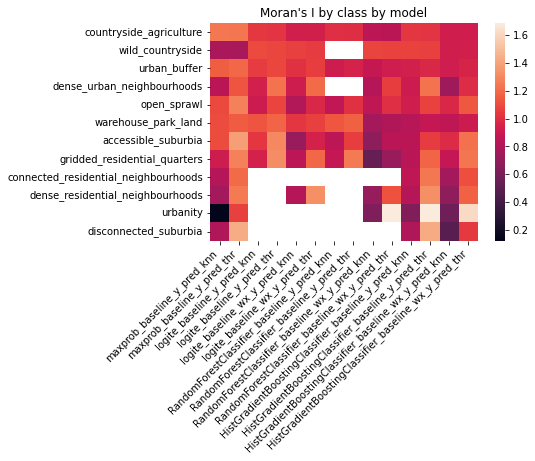IMPORANT - Note there are some NaN values in the table, these correspond to instances where a model does not predict any chip in a given class.

##### Joint counts¶

Here we look at joint counts and, specifically, focus on the proportion of pairs of a given class that are neighbors.

tst = tools.spatial_perf((sp_db['label'], w_knn, None))

tst

(                                         moran       jcs
urban_buffer                          0.650926  104942.5
countryside_agriculture               0.761201  109258.0
dense_urban_neighbourhoods            0.281699     358.0
open_sprawl                           0.464526   12172.0
gridded_residential_quarters          0.293207     440.0
accessible_suburbia                   0.303767    2649.5
warehouse_park_land                   0.718528    7098.5
connected_residential_neighbourhoods  0.153006     199.0
dense_residential_neighbourhoods      0.222241     414.5
disconnected_suburbia                 0.037901      15.0
urbanity                              0.045493       4.0
wild_countryside                      0.832364   13276.5,
None)